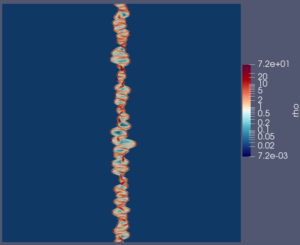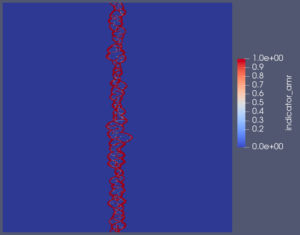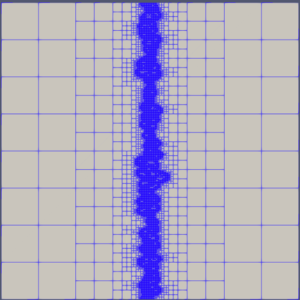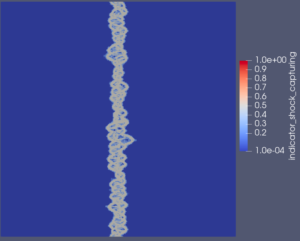# Snapshot: Simulation of a colliding flow setup with DGSEM

Simulation of a colliding flow setup with DGSEM (polynomial degree 3,
compatible sub-cell LGL type FV for shock capturing) of the compressible
Euler equations, where at x = +/- 64 inflow boundary conditions with
Mach number Ma = 70 is set (with periodic boundary conditions in y
direction).

Density distribution, where the color bar is plotted in logarithmic scale, at
final simulation time t=25:Simulation with AMR (level 3 up to level 9), showing the AMR indicator at
final time t=25:Plot of the DGSEM/FV blending factor for shock capturing at final time
t=25:The combination of the AMR indicator and the shock capturing blending
factor triggers the final mesh refinement, as can be seen here at the final time t=25: